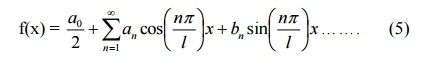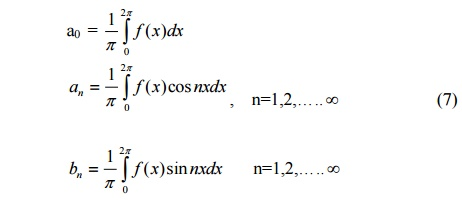Home | | Transforms and Partial Differential Equations | Formula For Fourier Series

# Formula For Fourier Series

Mathematics (maths) - Fourier Series - Formula For Fourier Series - Consider a real-valued function f(x) which obeys the following conditions called Dirichletâ€źs conditions

A Fourier series of a periodic function consists of a sum of sine and cosine terms. Sines and cosines are the most fundamental periodic functions.The Fourier series is named after the French Mathematician and Physicist Jacques Fourier (1768 â€“1830). Fourier series has its application in problems pertaining to Heat conduction, acoustics, etc. The subject matter may be divided into the following sub topics.FORMULA FOR FOURIER SERIES

Consider a real-valued function f(x) which obeys the following conditions called

Dirichletâ€źs conditions   :

1.     f(x) is defined in an interval (a,a+2l), and f(x+2l) = f(x) so that f(x) is a periodic function of period 2l

2.     f(x) is continuous or has only a finite number of discontinuities in the interval (a,a+2l).

3.     f(x) has no or only a finite number of maxima or minima in the interval (a,a+2l).

Also, letis called the Fourier series of f(x) in the interval (a,a+2l). Also, the real numbers a0, a1, a2, â€¦.an, and b1, b2 , â€¦.bare called the Fourier coefficients of f(x). The formulae (1), (2) and   (3)   are   called   Eulerâ€źs   formulae.

It can be proved that the sum of the series (4) is f(x) if f(x) is continuous at x. Thus we haveSuppose f(x) is discontinuous at x, then the sum of the series (4) wouldwhere f(x+) and f(x-) are the values of f(x) immediately to the right and to the left of f(x) respectively.

Particular Cases Case (i)

Suppose a=0. Then f(x) is defined over the interval (0,2l). Formulae (1), (2), (3) reduce toThen the right-hand side of (5) is the Fourier expansion of f(x) over the interval (0,2l).

If we set l=p, then f(x) is defined over the interval (0,2p). Formulae (6) reduce toAlso, in this case, (5) becomesCase (ii)

Suppose a=-l. Then f(x) is defined over the interval (-l , l). Formulae (1), (2) (3) reduce toThen the right-hand side of (5) is the Fourier expansion of f(x) over the interval (-l , l).

If we set l = p, then f(x) is defined over the interval (-pp).  Formulae (9) reduce toSome useful results :

1.     The   following   rule   called   Bernoulliâ€źseful in evaluating the Fourier coefficients.2.       The following integrals are also useful :3.                                         If   â€žnâ€ź   is   integer,   then

sin np= 0 ,       cosnp= (-1)n ,        sin2np= 0,  cos2np=1

Study Material, Lecturing Notes, Assignment, Reference, Wiki description explanation, brief detail
Mathematics (maths) : Fourier Series : Formula For Fourier Series |